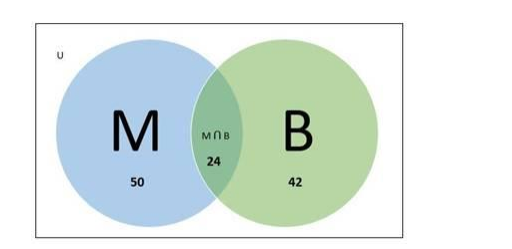# In a class of a certain school, 50 students, offered mathematics

Question:

In a class of a certain school, 50 students, offered mathematics, 42 offered biology and 24 offered both the subjects. Find the number of students offering

(i) mathematics only,

(ii) biology only,

(iii) any of the two subjects.

Solution:

Given:

Number of students offered Mathematics $=50$

Number of students offered Biology $=42$

Number of students offered both Mathematics and Biology $=24$

To Find:

(i) Number of students offered Mathematics only

Let us consider,

Number of students offered Mathematics $=n(M)=50$

Number of students offered Biology $=n(B)=42$

Number of students offered Mathematics \& Biology both $=n(M \cap B)=24$

Number of students offered Mathematics only $=n(M-B)$

Venn diagram:Now

$n(M-B)=n(M)-n(M \cap B)$

$=50-24$

$=26$

Therefore, Number of students offered Mathematics only= 26

(ii) Number of students offered Biology only

Number of students offered Biology only $=n(B-M)$

Now,

$n(B-M)=n(B)-n(M \cap B)$

$=42-24$

$=18$

Therefore, Number of students offered Biology only $=18$

(iii) Number of students offered any of two subjects

Number of students offered any of two subjects $=n(M \cup B)$

Now,

$n(M \cup B)=n(M)+n(B)-n(M \cap B)$

$=50+42-24$

$=140$

Therefore, Number of students offered any of two subjects = 68